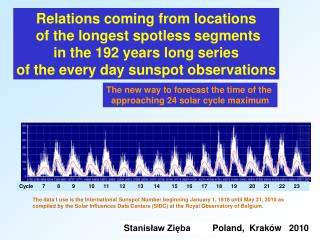DownloadDownload PresentationRelations coming from locations of the longest spotless segments in the 19 2 years long series of the every day suns

# Relations coming from locations of the longest spotless segments in the 19 2 years long series of the every day suns

Download Presentation## Relations coming from locations of the longest spotless segments in the 19 2 years long series of the every day suns

- - - - - - - - - - - - - - - - - - - - - - - - - - - E N D - - - - - - - - - - - - - - - - - - - - - - - - - - -
##### Presentation Transcript

1. Relations coming from locations of the longest spotless segments in the 192 years long series of the every day sunspot observations The new way to forecast the time of the approaching 24 solar cycle maximum Cycle 7 8 9 10 11 12 13 14 15 16 17 18 19 20 21 22 23 The data I use is the International Sunspot Number beginning January 1, 1818 until May 31, 2010 as compiled by the Solar Influences Data Centers (SIDC) at the Royal Observatory of Belgium. Stanisław ZiębaPoland, Kraków 2010

2. 47261 – the peak maximum 51127 – the peak maximum Cycle 19 Cycle 18 Transition region Nr 19 47614 – the Gauss maximum 51216 – the Gauss maximum 48566 - the first spotless day 50329 - the last spotless day 49842 – the day in the middle of the longest (30 days) spotless segment. Some definitions The transition region is defined as the distance (denoted as d00) from the first spotless day after a cycle maximum to the last spotless day before the next maximum. This is the same definition as it was use to define so called the passive interval of solar activity in our paper Zięba et. al. ApJ 653:1517-1530, 2006. The name transition region is probably more adequate. The transition region number is taken from the number of the cycle before which this region is chosen. Days are numerated from the January 1, 1818. The position of the longestspotless segment can be measured as a distance (ds0) from the first spotless day or as a distance from the chosen maximum. Two different definition of a maximum are recognised. The peak maximum is determined by the day with the maximal sunspot number while the Gauss maximum is described by the day in which values obtained from observations after filtering using the 1080 day Gauss filter (presented as the red line) has the maximal value. From the above defined points the following distances can be calculated: dpx, dGx – the distances between the two successive maximums, peak or 1080 Gauss, respectively; dp0, dG0 – the distances of the longest spotless segment from the left peak or 1080 Gauss maximum. For the presented fragment of the data: d00=1764 ; ds0=1277 ; dpx=3867 ; dGx=3603 ; dp0=2582 ; dG0=2229, which allows to calculate the three different ratio r00=ds0/d00=0.724 ; rp0=dp0/dpx=0.668 ; rG0=dG0/dGx=0.619

3. Relation between the position of the longest spotless region ds0 and the length of the transition region d00 Nr d00 ds0 r00 For the transition region 24 ds0=2028, so from the obtained relations d00=3106 (exponential formula), d00=3162 (linear formula). The last spotless day according to this calculation can be observed near the day (67961+3106=71067)that is in the middle of 2012. Of course, shifts about a year are possible in both directions.

4. Relations between the position of the longest spotlessregiondp0, dG0 and the distances dpx, dGx between the two successive maximums For the transition region 24, from the values dp0 and dG0, it is possible to calculate the time of the 24 cycle maximum [observed (peak) and the 1080 Gauss average] using the above relations. From dp0=3314, dpx=1.27•3314+728=4937, tpx24=66675+4937=71612; >>>> January 2014 dG0=3133, dGx=1.36•3133+672=4933, tGx24=66856+4933=71789; >>>> July 2014 Cycle 23 Transient region 24 69989 – the longest (32 days) spotless segment 1999 2000 2001 2002 2003 2004 2005 2006 2007 2008 2009

5. Variations of rpx and rGx with transition region Nr dp0 dG0 dpx dGx rpx rGx The inferred values dpx and dGx allow to calculate the ratios rpx and rGx. They are for the transition region 24: rpx=3314/4937=0.671 and rGx=3133/4933=0.635 The lowest values of rpx and rGx appear for transient regions 22 and 23 for which the largest differences betweenthe both discussed distances dpx and dGx are observed.